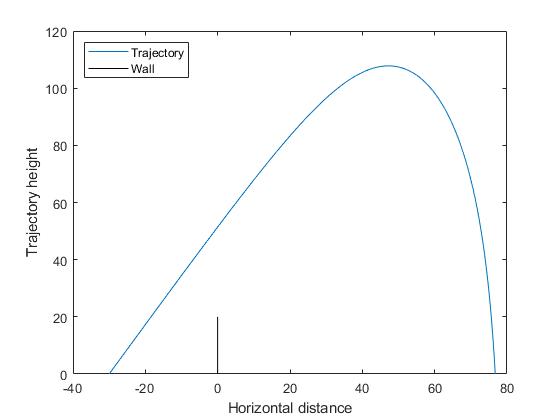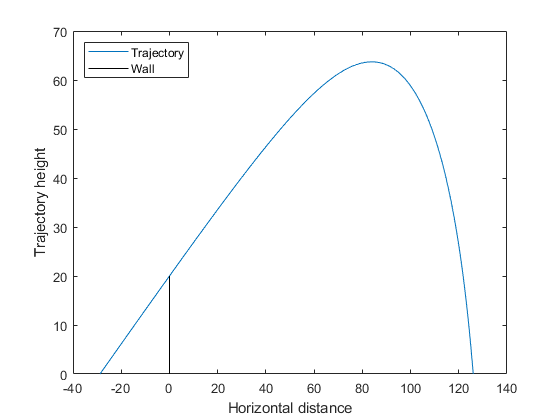## Optimize ODEs in Parallel

This example shows how to optimize parameters of an ODE.

It also shows how to avoid computing the objective and nonlinear constraint function twice when the ODE solution returns both. The example compares `patternsearch` and `ga` in terms of time to run the solver and the quality of the solutions.

You need a Parallel Computing Toolbox™ license to use parallel computing.

### Step 1. Define the problem.

The problem is to change the position and angle of a cannon to fire a projectile as far as possible beyond a wall. The cannon has a muzzle velocity of 300 m/s. The wall is 20 m high. If the cannon is too close to the wall, it has to fire at too steep an angle, and the projectile does not travel far enough. If the cannon is too far from the wall, the projectile does not travel far enough either.

Air resistance slows the projectile. The resisting force is proportional to the square of the velocity, with proportionality constant 0.02. Gravity acts on the projectile, accelerating it downward with constant 9.81 m/s2. Therefore, the equations of motion for the trajectory x(t) are

`$\frac{{d}^{2}x\left(t\right)}{d{t}^{2}}=-0.02‖v\left(t\right)‖v\left(t\right)-\left(0,9.81\right),$`

where $v\left(t\right)\text{​}=dx\left(t\right)/dt.$

The initial position `x0` and initial velocity `xp0` are 2-D vectors. However, the initial height `x0(2)` is 0, so the initial position depends only on the scalar `x0(1)`. And the initial velocity `xp0` has magnitude 300 (the muzzle velocity), so depends only on the initial angle, a scalar. For an initial angle `th`, `xp0` = `300*(cos(th),sin(th))`. Therefore, the optimization problem depends only on two scalars, so it is a 2-D problem. Use the horizontal distance and the angle as the decision variables.

### Step 2. Formulate the ODE model.

ODE solvers require you to formulate your model as a first-order system. Augment the trajectory vector (x1(t),x2(t)) with its time derivative (x'1(t),x'2(t)) to form a 4-D trajectory vector. In terms of this augmented vector, the differential equation becomes

`$\frac{d}{dt}x\left(t\right)=\left[\begin{array}{c}{x}_{3}\left(t\right)\\ {x}_{4}\left(t\right)\\ -.02‖\left({x}_{3}\left(t\right),{x}_{4}\left(t\right)\right)‖{x}_{3}\left(t\right)\\ -.02‖\left({x}_{3}\left(t\right),{x}_{4}\left(t\right)\right)‖{x}_{4}\left(t\right)-9.81\end{array}\right].$`

Write the differential equation as a function file, and save it on your MATLAB® path.

```function f = cannonfodder(t,x) f = [x(3);x(4);x(3);x(4)]; % Initial, gets f(1) and f(2) correct nrm = norm(x(3:4)) * .02; % Norm of the velocity times constant f(3) = -x(3)*nrm; % Horizontal acceleration f(4) = -x(4)*nrm - 9.81; % Vertical acceleration```

Visualize the solution of the ODE starting 30 m from the wall at an angle of `pi/3`.### Step 3. Solve using patternsearch.

The problem is to find initial position `x0(1)` and initial angle `x0(2)` to maximize the distance from the wall the projectile lands. Because this is a maximization problem, minimize the negative of the distance (see Maximizing vs. Minimizing).

To use `patternsearch` to solve this problem, you must provide the objective, constraint, initial guess, and options.

These two files are the objective and constraint functions. Copy them to a folder on your MATLAB path.

```function f = cannonobjective(x) x0 = [x(1);0;300*cos(x(2));300*sin(x(2))]; sol = ode45(@cannonfodder,[0,15],x0); % Find the time t when y_2(t) = 0 zerofnd = fzero(@(r)deval(sol,r,2),[sol.x(2),sol.x(end)]); % Then find the x-position at that time f = deval(sol,zerofnd,1); f = -f; % take negative of distance for maximization function [c,ceq] = cannonconstraint(x) ceq = []; x0 = [x(1);0;300*cos(x(2));300*sin(x(2))]; sol = ode45(@cannonfodder,[0,15],x0); if sol.y(1,end) <= 0 % Projectile never reaches wall c = 20 - sol.y(2,end); else % Find when the projectile crosses x = 0 zerofnd = fzero(@(r)deval(sol,r,1),[sol.x(2),sol.x(end)]); % Then find the height there, and subtract from 20 c = 20 - deval(sol,zerofnd,2); end```

Notice that the objective and constraint functions set their input variable `x0` to a 4-D initial point for the ODE solver. The ODE solver does not stop if the projectile hits the wall. Instead, the constraint function simply becomes positive, indicating an infeasible initial value.

The initial position `x0(1)` cannot be above 0, and it is futile to have it be below –200. (It should be near –20 because, with no air resistance, the longest trajectory would start at –20 at an angle `pi/4`.) Similarly, the initial angle `x0(2)` cannot be below 0, and cannot be above `pi/2`. Set bounds slightly away from these initial values:

```lb = [-200;0.05]; ub = [-1;pi/2-.05]; x0 = [-30,pi/3]; % Initial guess```

Set the `UseCompletePoll` option to `true`. This gives a higher-quality solution, and enables direct comparison with parallel processing, because computing in parallel requires this setting.

`opts = optimoptions('patternsearch','UseCompletePoll',true);`

Call `patternsearch` to solve the problem.

```tic % Time the solution [xsolution,distance,eflag,outpt] = patternsearch(@cannonobjective,x0,... [],[],[],[],lb,ub,@cannonconstraint,opts) toc```
```Optimization terminated: mesh size less than options.MeshTolerance and constraint violation is less than options.ConstraintTolerance. xsolution = -28.8123 0.6095 distance = -125.9880 eflag = 1 outpt = struct with fields: function: @cannonobjective problemtype: 'nonlinearconstr' pollmethod: 'gpspositivebasis2n' maxconstraint: 0 searchmethod: [] iterations: 5 funccount: 269 meshsize: 8.9125e-07 rngstate: [1×1 struct] message: 'Optimization terminated: mesh size less than options.MeshTolerance↵ and constraint violation is less than options.ConstraintTolerance.' Elapsed time is 0.792152 seconds.```

Starting the projectile about 29 m from the wall at an angle 0.6095 radian results in the farthest distance, about 126 m. The reported distance is negative because the objective function is the negative of the distance to the wall.

Visualize the solution.

```x0 = [xsolution(1);0;300*cos(xsolution(2));300*sin(xsolution(2))]; sol = ode45(@cannonfodder,[0,15],x0); % Find the time when the projectile lands zerofnd = fzero(@(r)deval(sol,r,2),[sol.x(2),sol.x(end)]); t = linspace(0,zerofnd); % equal times for plot xs = deval(sol,t,1); % Interpolated x values ys = deval(sol,t,2); % Interpolated y values plot(xs,ys) hold on plot([0,0],[0,20],'k') % Draw the wall xlabel('Horizontal distance') ylabel('Trajectory height') legend('Trajectory','Wall','Location','NW') ylim([0 70]) hold off```### Step 4. Avoid calling the expensive subroutine twice.

Both the objective and nonlinear constraint function call the ODE solver to calculate their values. Use the technique in Objective and Nonlinear Constraints in the Same Function to avoid calling the solver twice. The `runcannon` file implements this technique. Copy this file to a folder on your MATLAB path.

```function [x,f,eflag,outpt] = runcannon(x0,opts) if nargin == 1 % No options supplied opts = []; end xLast = []; % Last place ode solver was called sol = []; % ODE solution structure fun = @objfun; % The objective function, nested below cfun = @constr; % The constraint function, nested below lb = [-200;0.05]; ub = [-1;pi/2-.05]; % Call patternsearch [x,f,eflag,outpt] = patternsearch(fun,x0,[],[],[],[],lb,ub,cfun,opts); function y = objfun(x) if ~isequal(x,xLast) % Check if computation is necessary x0 = [x(1);0;300*cos(x(2));300*sin(x(2))]; sol = ode45(@cannonfodder,[0,15],x0); xLast = x; end % Now compute objective function % First find when the projectile hits the ground zerofnd = fzero(@(r)deval(sol,r,2),[sol.x(2),sol.x(end)]); % Then compute the x-position at that time y = deval(sol,zerofnd,1); y = -y; % take negative of distance end function [c,ceq] = constr(x) ceq = []; if ~isequal(x,xLast) % Check if computation is necessary x0 = [x(1);0;300*cos(x(2));300*sin(x(2))]; sol = ode45(@cannonfodder,[0,15],x0); xLast = x; end % Now compute constraint functions % First find when the projectile crosses x = 0 zerofnd = fzero(@(r)deval(sol,r,1),[sol.x(1),sol.x(end)]); % Then find the height there, and subtract from 20 c = 20 - deval(sol,zerofnd,2); end end```

Reinitialize the problem and time the call to `runcannon`.

```x0 = [-30;pi/3]; tic [xsolution,distance,eflag,outpt] = runcannon(x0,opts); toc```
```Optimization terminated: mesh size less than options.MeshTolerance and constraint violation is less than options.ConstraintTolerance. Elapsed time is 0.670715 seconds.```

The solver ran faster than before. If you examine the solution, you see that the output is identical.

### Step 5. Compute in parallel.

Try to save more time by computing in parallel. Begin by opening a parallel pool of workers.

`parpool`
```Starting parpool using the 'local' profile ... Connected to the parallel pool (number of workers: 6). ans = ProcessPool with properties: Connected: true NumWorkers: 6 Cluster: local AttachedFiles: {} AutoAddClientPath: true IdleTimeout: 30 minutes (30 minutes remaining) SpmdEnabled: true```

Set the options to use parallel computing, and rerun the solver.

```opts = optimoptions('patternsearch',opts,'UseParallel',true); x0 = [-30;pi/3]; tic [xsolution,distance,eflag,outpt] = runcannon(x0,opts); toc```
```Optimization terminated: mesh size less than options.MeshTolerance and constraint violation is less than options.ConstraintTolerance. Elapsed time is 1.894515 seconds.```

In this case, parallel computing was slower. If you examine the solution, you see that the output is identical.

### Step 6. Compare with the genetic algorithm.

You can also try to solve the problem using the genetic algorithm. However, the genetic algorithm is usually slower and less reliable.

As explained in Objective and Nonlinear Constraints in the Same Function, you cannot save time when using `ga` by the nested function technique used by `patternsearch` in Step 4. Instead, call `ga` in parallel by setting the appropriate options.

```options = optimoptions('ga','UseParallel',true); rng default % For reproducibility tic % Time the solution [xsolution,distance,eflag,outpt] = ga(@cannonobjective,2,... [],[],[],[],lb,ub,@cannonconstraint,options) toc```
```Optimization terminated: average change in the fitness value less than options.FunctionTolerance and constraint violation is less than options.ConstraintTolerance. xsolution = -37.6217 0.4926 distance = -122.2181 eflag = 1 outpt = struct with fields: problemtype: 'nonlinearconstr' rngstate: [1×1 struct] generations: 4 funccount: 9874 message: 'Optimization terminated: average change in the fitness value less than options.FunctionTolerance↵ and constraint violation is less than options.ConstraintTolerance.' maxconstraint: 0 hybridflag: [] Elapsed time is 12.529131 seconds.```

The `ga` solution is not as good as the `patternsearch` solution: 122 m versus 126 m. `ga` takes more time: about 12 s versus under 2 s; `patternsearch` takes even less time in serial and nested, less than 1 s. Running `ga` serially takes even longer, about 30 s in one test run.